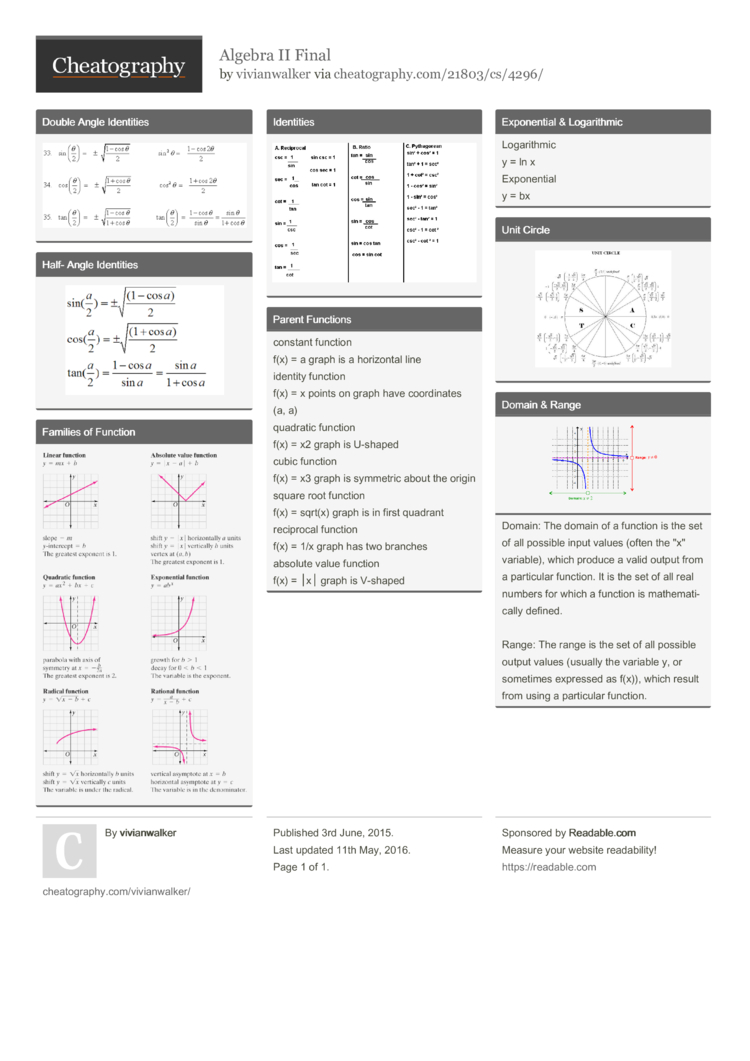# Algebra II Final by vivianwalker

Alg. II Final

### Double Angle Identities### Half- Angle Identities### Families of Function### Identities### Parent Functions

 constant function f(x) = a graph is a horizontal line identity function f(x) = x points on graph have coordi­­nates (a, a) quadratic function f(x) = x2 graph is U-shaped cubic function f(x) = x3 graph is symmetric about the origin square root function f(x) = sqrt(x) graph is in first quadrant reciprocal function f(x) = 1/x graph has two branches absolute value function f(x) = │x│ graph is V-shaped

### Expone­ntial & Logari­thmic

 Logari­­thmic y = ln x Expone­­ntial y = bx

### Unit Circle### Domain & RangeDoma­in: The domain of a function is the set of all possible input values (often the "­­­x­" variable), which produce a valid output from a particular function. It is the set of all real numbers for which a function is mathem­­­a­t­i­­cally defined.

Range: The range is the set of all possible output values (usually the variable y, or sometimes expressed as f(x)), which result from using a particular function.1 Page
//media.cheatography.com/storage/thumb/vivianwalker_algebra-ii-final.750.jpg

PDF (recommended)